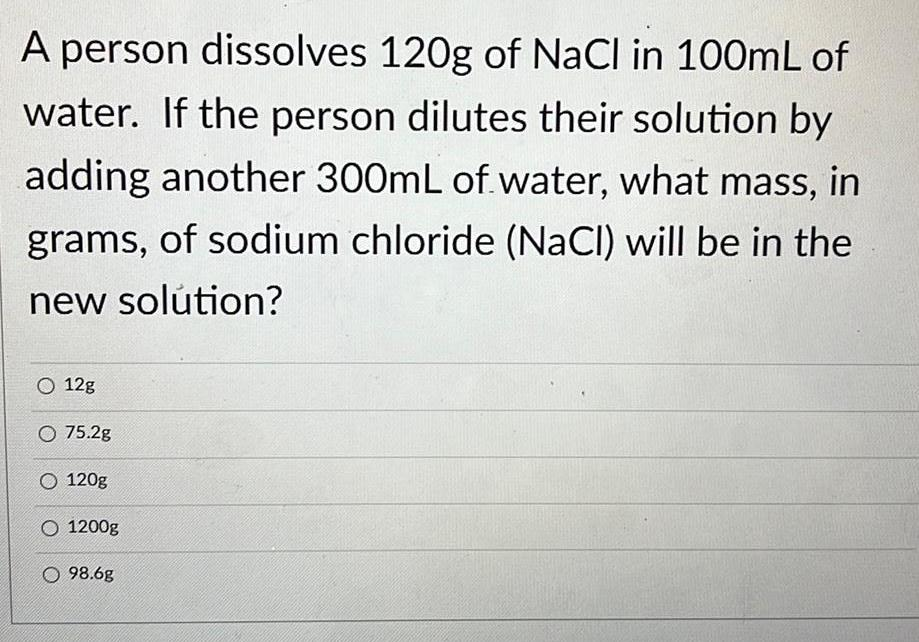Organic Chemistry
Polymers
A person dissolves 120g of NaCl in 100mL of water. If the person dilutes their solution by adding another 300mL of water, what mass, in grams, of sodium chloride (NaCl) will be in the new solution? 12g 75.2g 120g 1200g 98.6g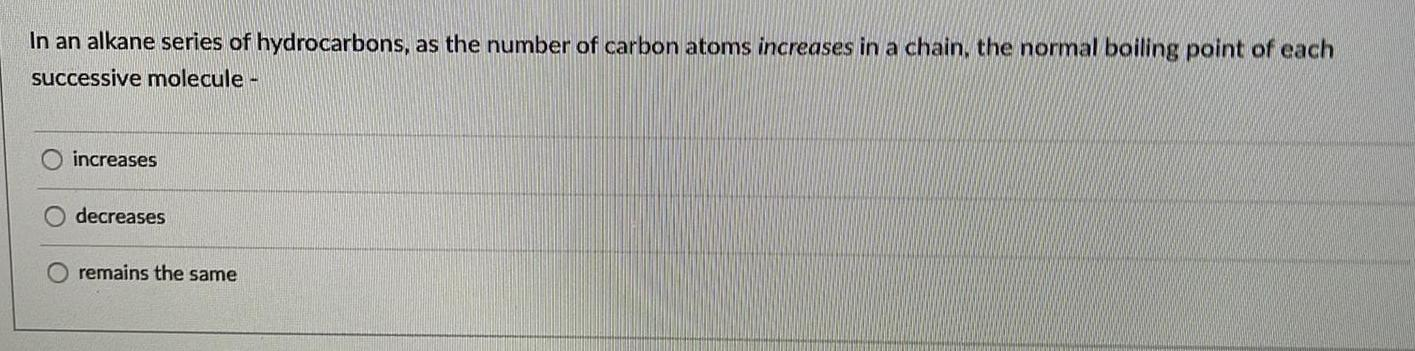Organic Chemistry
Polymers
In an alkane series of hydrocarbons, as the number of carbon atoms increases in a chain, the normal boiling point of each successive molecule - increases decreases remains the same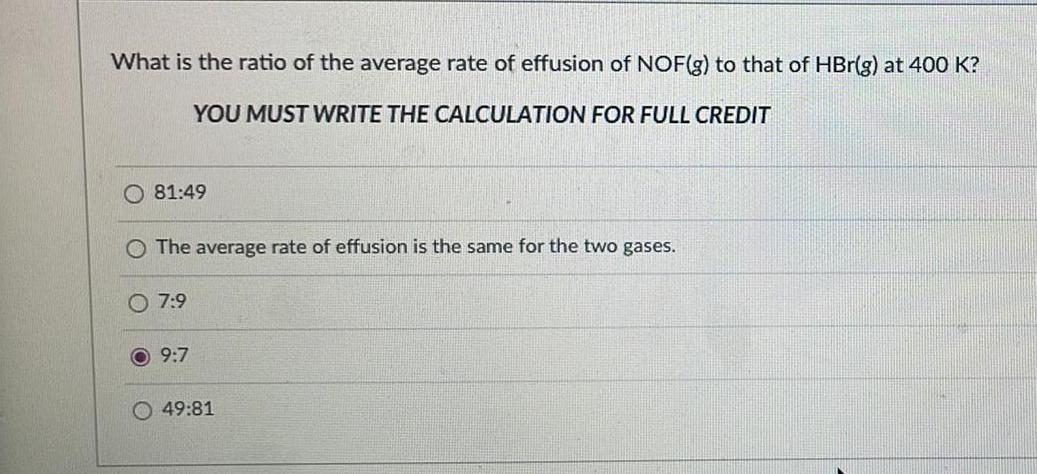Organic Chemistry
Polymers
What is the ratio of the average rate of effusion of NOF(g) to that of HBr(g) at 400 K? YOU MUST WRITE THE CALCULATION FOR FULL CREDIT 81:49 The average rate of effusion is the same for the two gases. 7:9 9:7 49:81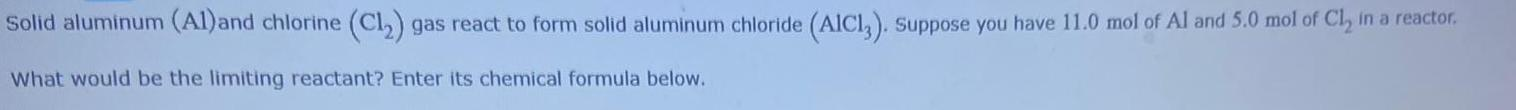Organic Chemistry
Polymers
Solid aluminum (Al) and chlorine (C1₂) gas react to form solid aluminum chloride (AIC13). Suppose you have 11.0 mol of Al and 5.0 mol of Cl₂ in a reactor. What would be the limiting reactant? Enter its chemical formula below.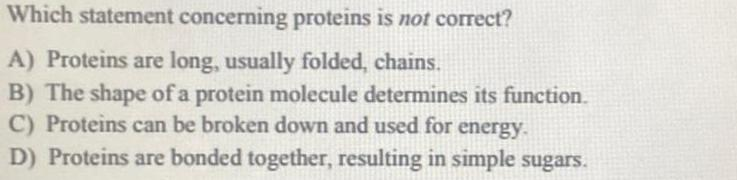Organic Chemistry
Polymers
Which statement concerning proteins is not correct? A) Proteins are long, usually folded, chains. B) The shape of a protein molecule determines its function. C) Proteins can be broken down and used for energy. D) Proteins are bonded together, resulting in simple sugars.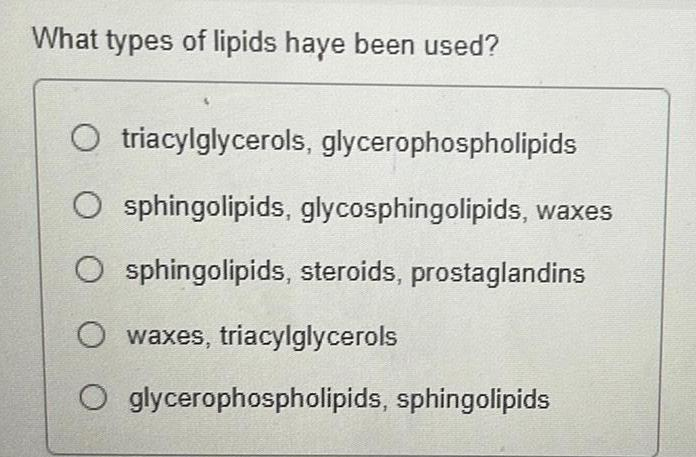Organic Chemistry
Polymers
What types of lipids have been used? triacylglycerols, glycerophospholipids sphingolipids, glycosphingolipids, waxes sphingolipids, steroids, prostaglandins waxes, triacylglycerols glycerophospholipids, sphingolipids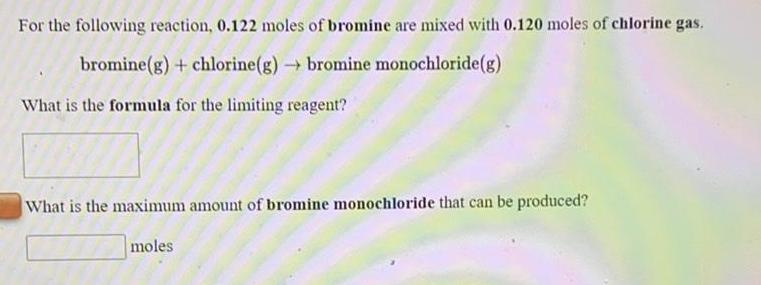Organic Chemistry
Polymers
For the following reaction, 0.122 moles of bromine are mixed with 0.120 moles of chlorine gas. bromine(g) + chlorine(g) → bromine monochloride (g) What is the formula for the limiting reagent? What is the maximum amount of bromine monochloride that can be produced?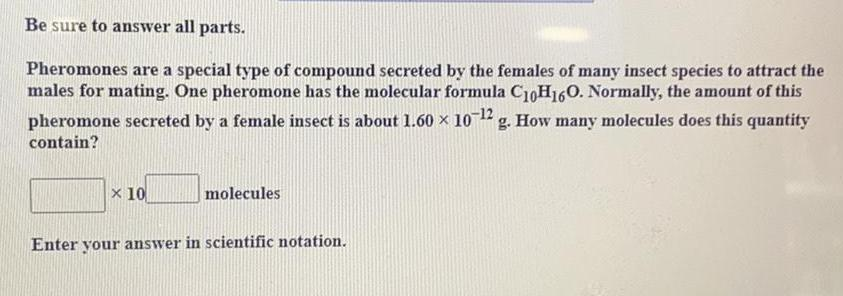Organic Chemistry
Polymers
Pheromones are a special type of compound secreted by the females of many insect species to attract the males for mating. One pheromone has the molecular formula C10H16O. Normally, the amount of this pheromone secreted by a female insect is about 1.60 × 10^-12 g. How many molecules does this quantity contain? Enter your answer in scientific notation.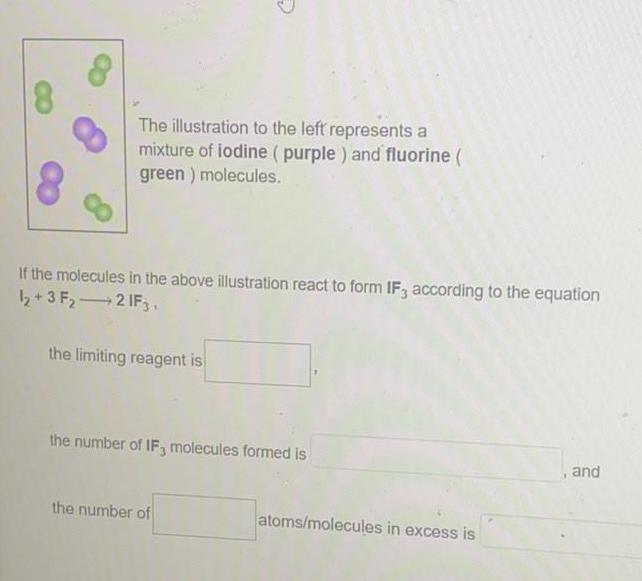Organic Chemistry
Polymers
The illustration to the left represents a mixture of iodine (purple) and fluorine ( green) molecules. If the molecules in the above illustration react to form IF3 according to the equation I2+3 F₂ ---> 2 IF3 the limiting reagent is the number of IF3 molecules formed is, and the number of atoms/molecules in excess is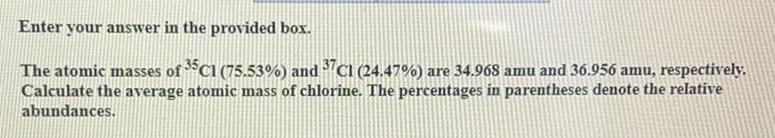Organic Chemistry
Polymers
The atomic masses of 35C1 (75.53%) and 37C1 (24.47%) are 34.968 amu and 36.956 amu, respectively. Calculate the average atomic mass of chlorine. The percentages in parentheses denote the relative abundances.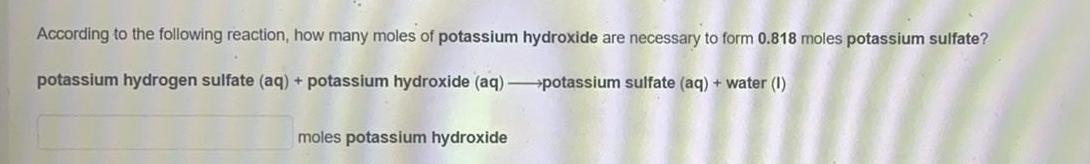Organic Chemistry
Polymers
According to the following reaction, how many moles of potassium hydroxide are necessary to form 0.818 moles potassium sulfate? potassium hydrogen sulfate (aq) + potassium hydroxide (aq) ---> potassium sulfate (aq) + water(l)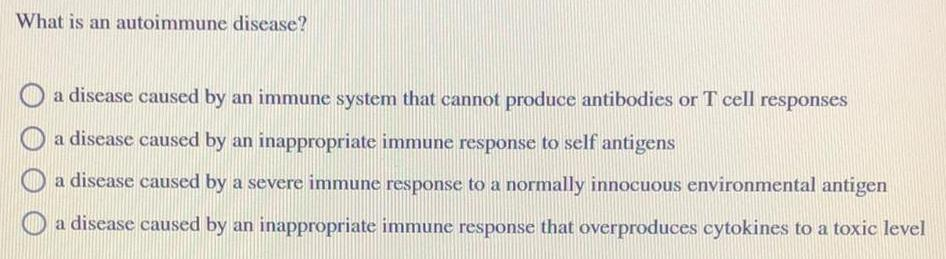Organic Chemistry
Polymers
What is an autoimmune disease? a disease caused by an immune system that cannot produce antibodies or T cell responses a disease caused by an inappropriate immune response to self antigens a disease caused by a severe immune response to a normally innocuous environmental antigen a disease caused by an inappropriate immune response that overproduces cytokines to a toxic level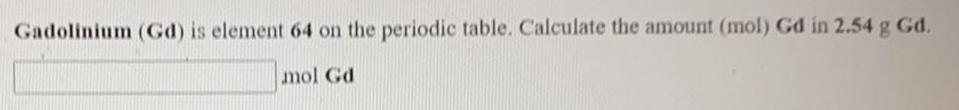Organic Chemistry
Polymers
Gadolinium (Gd) is element 64 on the periodic table. Calculate the amount (mol) Gd in 2.54 g Gd.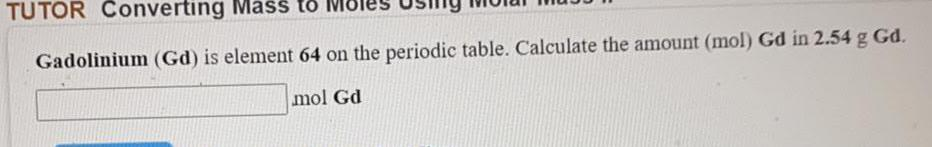Organic Chemistry
Polymers
Gadolinium (Gd) is element 64 on the periodic table. Calculate the amount (mol) Gd in 2.54 g Gd.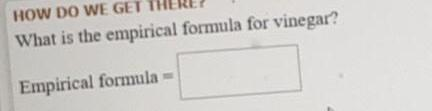Organic Chemistry
Polymers
What is the empirical formula for vinegar?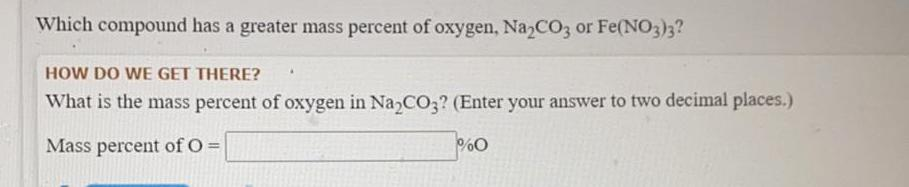Organic Chemistry
Polymers
Which compound has a greater mass percent of oxygen, Na₂CO3 or Fe(NO3)3? What is the mass percent of oxygen in Na₂CO3? (Enter your answer to two decimal places.) Mass percent of O =